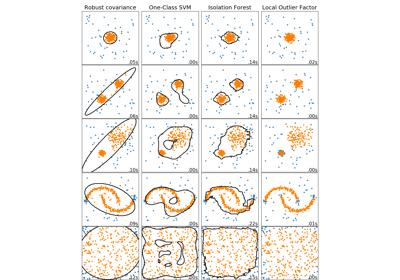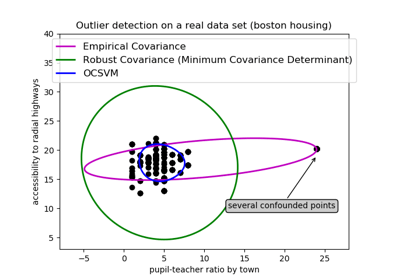/scikit-learn

# sklearn.covariance.EllipticEnvelope

`class sklearn.covariance.EllipticEnvelope(store_precision=True, assume_centered=False, support_fraction=None, contamination=0.1, random_state=None)` [source]

An object for detecting outliers in a Gaussian distributed dataset.

Read more in the User Guide.

Parameters: `store_precision : boolean, optional (default=True)` Specify if the estimated precision is stored. `assume_centered : boolean, optional (default=False)` If True, the support of robust location and covariance estimates is computed, and a covariance estimate is recomputed from it, without centering the data. Useful to work with data whose mean is significantly equal to zero but is not exactly zero. If False, the robust location and covariance are directly computed with the FastMCD algorithm without additional treatment. `support_fraction : float in (0., 1.), optional (default=None)` The proportion of points to be included in the support of the raw MCD estimate. If None, the minimum value of support_fraction will be used within the algorithm: `[n_sample + n_features + 1] / 2`. `contamination : float in (0., 0.5), optional (default=0.1)` The amount of contamination of the data set, i.e. the proportion of outliers in the data set. `random_state : int, RandomState instance or None, optional (default=None)` The seed of the pseudo random number generator to use when shuffling the data. If int, random_state is the seed used by the random number generator; If RandomState instance, random_state is the random number generator; If None, the random number generator is the RandomState instance used by `np.random`. `location_ : array-like, shape (n_features,)` Estimated robust location `covariance_ : array-like, shape (n_features, n_features)` Estimated robust covariance matrix `precision_ : array-like, shape (n_features, n_features)` Estimated pseudo inverse matrix. (stored only if store_precision is True) `support_ : array-like, shape (n_samples,)` A mask of the observations that have been used to compute the robust estimates of location and shape. `offset_ : float` Offset used to define the decision function from the raw scores. We have the relation: `decision_function = score_samples - offset_`. The offset depends on the contamination parameter and is defined in such a way we obtain the expected number of outliers (samples with decision function < 0) in training.

#### Notes

Outlier detection from covariance estimation may break or not perform well in high-dimensional settings. In particular, one will always take care to work with `n_samples > n_features ** 2`.

#### References

  Rousseeuw, P.J., Van Driessen, K. “A fast algorithm for the minimum covariance determinant estimator” Technometrics 41(3), 212 (1999)

#### Methods

 `correct_covariance`(data) Apply a correction to raw Minimum Covariance Determinant estimates. `decision_function`(X[, raw_values]) Compute the decision function of the given observations. `error_norm`(comp_cov[, norm, scaling, squared]) Computes the Mean Squared Error between two covariance estimators. `fit`(X[, y]) Fit the EllipticEnvelope model. `fit_predict`(X[, y]) Performs outlier detection on X. `get_params`([deep]) Get parameters for this estimator. `get_precision`() Getter for the precision matrix. `mahalanobis`(X) Computes the squared Mahalanobis distances of given observations. `predict`(X) Predict the labels (1 inlier, -1 outlier) of X according to the fitted model. `reweight_covariance`(data) Re-weight raw Minimum Covariance Determinant estimates. `score`(X, y[, sample_weight]) Returns the mean accuracy on the given test data and labels. `score_samples`(X) Compute the negative Mahalanobis distances. `set_params`(**params) Set the parameters of this estimator.
`__init__(store_precision=True, assume_centered=False, support_fraction=None, contamination=0.1, random_state=None)` [source]
`correct_covariance(data)` [source]

Apply a correction to raw Minimum Covariance Determinant estimates.

Correction using the empirical correction factor suggested by Rousseeuw and Van Driessen in [RVD].

Parameters: `data : array-like, shape (n_samples, n_features)` The data matrix, with p features and n samples. The data set must be the one which was used to compute the raw estimates. `covariance_corrected : array-like, shape (n_features, n_features)` Corrected robust covariance estimate.

#### References

 [RVD] (1, 2) `A Fast Algorithm for the Minimum Covariance Determinant Estimator, 1999, American Statistical Association and the American Society for Quality, TECHNOMETRICS`
`decision_function(X, raw_values=None)` [source]

Compute the decision function of the given observations.

Parameters: `X : array-like, shape (n_samples, n_features)` `raw_values : bool, optional` Whether or not to consider raw Mahalanobis distances as the decision function. Must be False (default) for compatibility with the others outlier detection tools. Deprecated since version 0.20: `raw_values` has been deprecated in 0.20 and will be removed in 0.22. `decision : array-like, shape (n_samples, )` Decision function of the samples. It is equal to the shifted Mahalanobis distances. The threshold for being an outlier is 0, which ensures a compatibility with other outlier detection algorithms.
`error_norm(comp_cov, norm=’frobenius’, scaling=True, squared=True)` [source]

Computes the Mean Squared Error between two covariance estimators. (In the sense of the Frobenius norm).

Parameters: `comp_cov : array-like, shape = [n_features, n_features]` The covariance to compare with. `norm : str` The type of norm used to compute the error. Available error types: - ‘frobenius’ (default): sqrt(tr(A^t.A)) - ‘spectral’: sqrt(max(eigenvalues(A^t.A)) where A is the error `(comp_cov - self.covariance_)`. `scaling : bool` If True (default), the squared error norm is divided by n_features. If False, the squared error norm is not rescaled. `squared : bool` Whether to compute the squared error norm or the error norm. If True (default), the squared error norm is returned. If False, the error norm is returned. The Mean Squared Error (in the sense of the Frobenius norm) between `self` and `comp_cov` covariance estimators.
`fit(X, y=None)` [source]

Fit the EllipticEnvelope model.

Parameters: `X : numpy array or sparse matrix, shape (n_samples, n_features).` Training data `y : Ignored` not used, present for API consistency by convention.
`fit_predict(X, y=None)` [source]

Performs outlier detection on X.

Returns -1 for outliers and 1 for inliers.

Parameters: `X : ndarray, shape (n_samples, n_features)` Input data. `y : Ignored` not used, present for API consistency by convention. `y : ndarray, shape (n_samples,)` 1 for inliers, -1 for outliers.
`get_params(deep=True)` [source]

Get parameters for this estimator.

Parameters: `deep : boolean, optional` If True, will return the parameters for this estimator and contained subobjects that are estimators. `params : mapping of string to any` Parameter names mapped to their values.
`get_precision()` [source]

Getter for the precision matrix.

Returns: `precision_ : array-like` The precision matrix associated to the current covariance object.
`mahalanobis(X)` [source]

Computes the squared Mahalanobis distances of given observations.

Parameters: `X : array-like, shape = [n_samples, n_features]` The observations, the Mahalanobis distances of the which we compute. Observations are assumed to be drawn from the same distribution than the data used in fit. `dist : array, shape = [n_samples,]` Squared Mahalanobis distances of the observations.
`predict(X)` [source]

Predict the labels (1 inlier, -1 outlier) of X according to the fitted model.

Parameters: `X : array-like, shape (n_samples, n_features)` `is_inlier : array, shape (n_samples,)` Returns -1 for anomalies/outliers and +1 for inliers.
`reweight_covariance(data)` [source]

Re-weight raw Minimum Covariance Determinant estimates.

Re-weight observations using Rousseeuw’s method (equivalent to deleting outlying observations from the data set before computing location and covariance estimates) described in [RVDriessen].

Parameters: `data : array-like, shape (n_samples, n_features)` The data matrix, with p features and n samples. The data set must be the one which was used to compute the raw estimates. `location_reweighted : array-like, shape (n_features, )` Re-weighted robust location estimate. `covariance_reweighted : array-like, shape (n_features, n_features)` Re-weighted robust covariance estimate. `support_reweighted : array-like, type boolean, shape (n_samples,)` A mask of the observations that have been used to compute the re-weighted robust location and covariance estimates.

#### References

 [RVDriessen] (1, 2) `A Fast Algorithm for the Minimum Covariance Determinant Estimator, 1999, American Statistical Association and the American Society for Quality, TECHNOMETRICS`
`score(X, y, sample_weight=None)` [source]

Returns the mean accuracy on the given test data and labels.

In multi-label classification, this is the subset accuracy which is a harsh metric since you require for each sample that each label set be correctly predicted.

Parameters: `X : array-like, shape (n_samples, n_features)` Test samples. `y : array-like, shape (n_samples,) or (n_samples, n_outputs)` True labels for X. `sample_weight : array-like, shape (n_samples,), optional` Sample weights. `score : float` Mean accuracy of self.predict(X) wrt. y.
`score_samples(X)` [source]

Compute the negative Mahalanobis distances.

Parameters: `X : array-like, shape (n_samples, n_features)` `negative_mahal_distances : array-like, shape (n_samples, )` Opposite of the Mahalanobis distances.
`set_params(**params)` [source]

Set the parameters of this estimator.

The method works on simple estimators as well as on nested objects (such as pipelines). The latter have parameters of the form `<component>__<parameter>` so that it’s possible to update each component of a nested object.

Returns: self

## Examples using `sklearn.covariance.EllipticEnvelope`Comparing anomaly detection algorithms for outlier detection on toy datasetsOutlier detection on a real data set